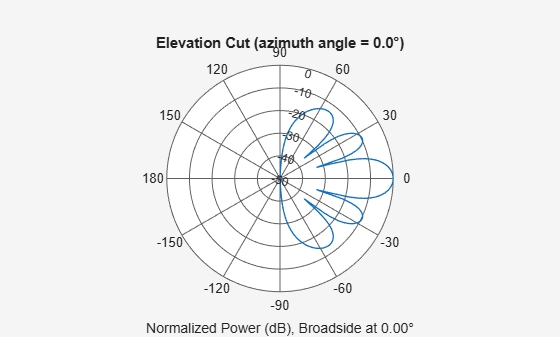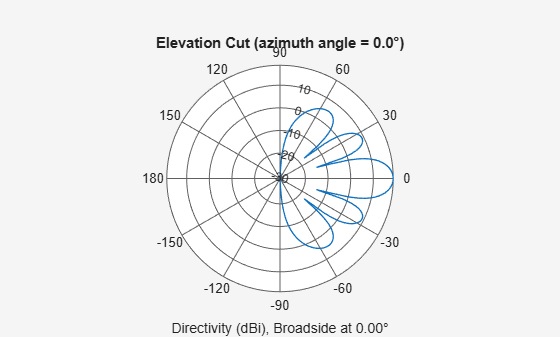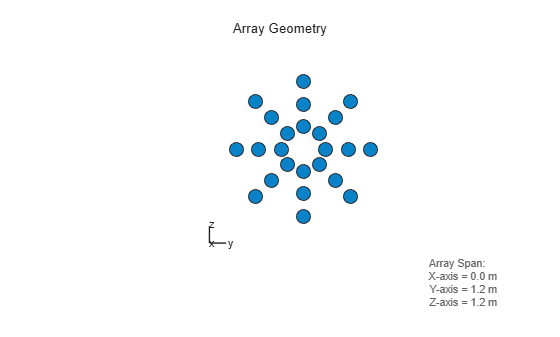# plotResponse

System object: phased.HeterogeneousConformalArray
Package: phased

Plot response pattern of array

## Syntax

```plotResponse(H,FREQ,V) plotResponse(H,FREQ,V,Name,Value) hPlot = plotResponse(___) ```

## Description

`plotResponse(H,FREQ,V)` plots the array response pattern along the azimuth cut, where the elevation angle is 0. The operating frequency is specified in `FREQ`. The propagation speed is specified in `V`.

`plotResponse(H,FREQ,V,Name,Value)` plots the array response with additional options specified by one or more `Name,Value` pair arguments.

`hPlot = plotResponse(___)` returns handles of the lines or surface in the figure window, using any of the input arguments in the previous syntaxes.

## Input Arguments

 `H` Array object `FREQ` Operating frequency in Hertz specified as a scalar or 1-by-K row vector. Values must lie within the range specified by a property of `H`. That property is named `FrequencyRange` or `FrequencyVector`, depending on the type of element in the array. The element has no response at frequencies outside that range. If you set the `'RespCut'` property of `H` to `'3D'`, `FREQ` must be a scalar. When `FREQ` is a row vector, `plotResponse` draws multiple frequency responses on the same axes. `V` Propagation speed in meters per second.

### Name-Value Pair Arguments

Specify optional comma-separated pairs of `Name,Value` arguments. `Name` is the argument name and `Value` is the corresponding value. `Name` must appear inside quotes. You can specify several name and value pair arguments in any order as `Name1,Value1,...,NameN,ValueN`.

`'CutAngle'`

Cut angle as a scalar. This argument is applicable only when `RespCut` is `'Az'` or `'El'`. If `RespCut` is `'Az'`, `CutAngle` must be between –90 and 90. If `RespCut` is `'El'`, `CutAngle` must be between –180 and 180.

Default: `0`

`'Format'`

Format of the plot, using one of `'Line'`, `'Polar'`, or `'UV'`. If you set `Format` to `'UV'`, `FREQ` must be a scalar.

Default: `'Line'`

`'NormalizeResponse'`

Set this value to `true` to normalize the response pattern. Set this value to `false` to plot the response pattern without normalizing it. This parameter is not applicable when you set the `Unit` parameter value to `'dbi'`.

Default: `true`

`'OverlayFreq'`

Set this value to `true` to overlay pattern cuts in a 2-D line plot. Set this value to `false` to plot pattern cuts against frequency in a 3-D waterfall plot. If this value is `false`, `FREQ` must be a vector with at least two entries.

This parameter applies only when `Format` is not `'Polar'` and RespCut is not `'3D'`.

Default: `true`

`'Polarization'`

Specify the polarization options for plotting the array response pattern. The allowable values are ```|'None' | 'Combined' | 'H' | 'V' |``` where

• `'None'` specifies plotting a nonpolarized response pattern

• `'Combined'` specifies plotting a combined polarization response pattern

• `'H'` specifies plotting the horizontal polarization response pattern

• `'V'` specifies plotting the vertical polarization response pattern

For arrays that do not support polarization, the only allowed value is `'None'`. This parameter is not applicable when you set the `Unit` parameter value to `'dbi'`.

Default: `'None'`

`'RespCut'`

Cut of the response. Valid values depend on `Format`, as follows:

• If `Format` is `'Line'` or `'Polar'`, the valid values of `RespCut` are `'Az'`, `'El'`, and `'3D'`. The default is `'Az'`.

• If `Format` is `'UV'`, the valid values of `RespCut` are `'U'` and `'3D'`. The default is `'U'`.

If you set `RespCut` to `'3D'`, `FREQ` must be a scalar.

`'Unit'`

The unit of the plot. Valid values are `'db'`, `'mag'`, `'pow'`, or `'dbi'`. This parameter determines the type of plot that is produced.

Unit valuePlot type
`db`power pattern in dB scale
`mag`field pattern
`pow`power pattern
`dbi`directivity

Default: `'db'`

`'Weights'`

Weight values applied to the array, specified as a length-N column vector or N-by-M matrix. The dimension N is the number of elements in the array. The interpretation of M depends upon whether the input argument `FREQ` is a scalar or row vector.

Weights DimensionsFREQ DimensionPurpose
N-by-1 column vectorScalar or 1-by-M row vectorApply one set of weights for the same single frequency or all M frequencies.
N-by-M matrixScalarApply all of the M different columns in `Weights` for the same single frequency.
1-by-M row vectorApply each of the M different columns in `Weights` for the corresponding frequency in `FREQ`.

`'AzimuthAngles'`

Azimuth angles for plotting array response, specified as a row vector. The `AzimuthAngles` parameter sets the display range and resolution of azimuth angles for visualizing the radiation pattern. This parameter is allowed only when the `RespCut` parameter is set to `'Az'` or `'3D'` and the `Format` parameter is set to `'Line'` or `'Polar'`. The values of azimuth angles should lie between –180° and 180° and must be in nondecreasing order. When you set the `RespCut` parameter to `'3D'`, you can set the `AzimuthAngles` and `ElevationAngles` parameters simultaneously.

Default: `[-180:180]`

`'ElevationAngles'`

Elevation angles for plotting array response, specified as a row vector. The `ElevationAngles` parameter sets the display range and resolution of elevation angles for visualizing the radiation pattern. This parameter is allowed only when the `RespCut` parameter is set to `'El'` or `'3D'` and the `Format` parameter is set to `'Line'` or `'Polar'`. The values of elevation angles should lie between –90° and 90° and must be in nondecreasing order. When yous set the `RespCut` parameter to `'3D'`, you can set the `ElevationAngles` and `AzimuthAngles` parameters simultaneously.

Default: `[-90:90]`

`'UGrid'`

U coordinate values for plotting array response, specified as a row vector. The `UGrid` parameter sets the display range and resolution of the U coordinates for visualizing the radiation pattern in U/V space. This parameter is allowed only when the `Format` parameter is set to `'UV'` and the `RespCut` parameter is set to `'U'` or `'3D'`. The values of `UGrid` should be between –1 and 1 and should be specified in nondecreasing order. You can set the `UGrid` and `VGrid` parameters simultaneously.

Default: `[-1:0.01:1]`

`'VGrid'`

V coordinate values for plotting array response, specified as a row vector. The `VGrid` parameter sets the display range and resolution of the V coordinates for visualizing the radiation pattern in U/V space. This parameter is allowed only when the `Format` parameter is set to `'UV'` and the `RespCut` parameter is set to `'3D'`. The values of `VGrid` should be between –1 and 1 and should be specified in nondecreasing order. You can set `VGrid` and `UGrid` parameters simultaneously.

Default: `[-1:0.01:1]`

## Examples

expand all

This example shows how to construct an 8-element uniform circular array (UCA) with two different antenna patterns.

```element1 = phased.CosineAntennaElement('CosinePower',1.5); element2 = phased.CosineAntennaElement('CosinePower',1.8); N = 8; azang = (0:N-1)*360/N-180; array = phased.HeterogeneousConformalArray( ... 'ElementPosition',0.4*[zeros(1,N); cosd(azang); sind(azang)], ... 'ElementNormal',zeros(2,N),'ElementSet',{element1,element2}, ... 'ElementIndices',[1 1 1 1 2 2 2 2]);```

Plot the array elevation response when the operating frequency is 1 GHz and the wave propagation speed is the speed of light.

```c = physconst('LightSpeed'); fc = 1e9; pattern(array,fc,0.0,-90:90,'PropagationSpeed',c,'CoordinateSystem','polar', ... 'Type','powerdb')```Plot the directivity.

```pattern(array,fc,0.0,-90:90,'PropagationSpeed',c,'CoordinateSystem','polar', ... 'Type','directivity')```This example shows how to construct a 24-element disk array using elements with two different antenna patterns and plot its response.

```sElement1 = phased.CosineAntennaElement('CosinePower',1.5); sElement2 = phased.CosineAntennaElement('CosinePower',1.8); N = 8; azang = (0:N-1)*360/N-180; p0 = [zeros(1,N);cosd(azang);sind(azang)]; posn = [0.6*p0, 0.4*p0, 0.2*p0]; sArray1 = phased.HeterogeneousConformalArray(... 'ElementPosition',posn,... 'ElementNormal', zeros(2,3*N),... 'ElementSet',{sElement1,sElement2},... 'ElementIndices',[1 1 1 1 1 1 1 1,... 1 1 1 1 1 1 1 1,... 2 2 2 2 2 2 2 2]);```

Show the array.

`viewArray(sArray1);`Plot the elevation response of this array using uniform weights on the elements and also a tapered set of weights set by the `Weights` parameter. Using the `ElevationAngles` parameter, restrict the plot of the response from -60 to 60 degrees in 0.1 degree increments. Assume the operating frequency is 1 GHz and the wave propagation speed is the speed of light.

```c = physconst('LightSpeed'); fc = 1e9; wts1 = ones(3*N,1); wts1 = wts1/sum(abs(wts1)); wts2 = [0.5*ones(N,1); 0.7*ones(N,1); 1*ones(N,1)]; wts2 = wts2/sum(abs(wts2)); plotResponse(sArray1,fc,c,'RespCut','El',... 'Format','Polar',... 'ElevationAngles',[-60:0.1:60],... 'Weights',... [wts1,wts2],... 'Unit','db');```As expected, the tapered weights broaden the mainlobe and reduce the sidelobes.